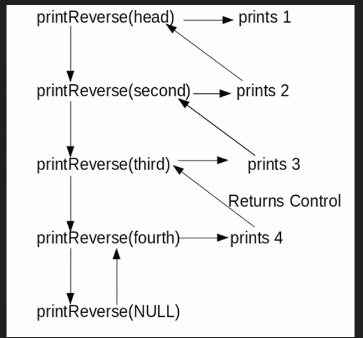# C Program For Printing Reverse Of A Linked List Without Actually Reversing

• Last Updated : 08 Jul, 2022

Given a linked list, print reverse of it using a recursive function. For example, if the given linked list is 1->2->3->4, then output should be 4->3->2->1.
Note that the question is only about printing the reverse. To reverse the list itself see this
Difficulty Level: RookieAlgorithm:

```printReverse(head)
1. call print reverse for head->next

Implementation:

## C

 `// C program to print reverse ` `// of a linked list` `#include` `#include` ` `  `// Link list node ` `struct` `Node` `{` `    ``int` `data;` `    ``struct` `Node* next;` `};` ` `  `// Function to reverse the linked list ` `void` `printReverse(``struct` `Node* head)` `{` `    ``// Base case  ` `    ``if` `(head == NULL)` `       ``return``;`   `    ``// Print the list after head node` `    ``printReverse(head->next);`   `    ``// After everything else is printed,` `    ``// print head` `    ``printf``(``"%d  "``, head->data);` `}` ` `  `// UTILITY FUNCTIONS` `/* Push a node to linked list. ` `   ``Note that this function` `   ``changes the head */` `void` `push(``struct` `Node** head_ref, ` `          ``char` `new_data)` `{` `    ``// Allocate node ` `    ``struct` `Node* new_node =` `           ``(``struct` `Node*) ``malloc``(``sizeof``(``struct` `Node));` ` `  `    ``// Put in the data  ` `    ``new_node->data  = new_data;` ` `  `    ``// Link the old list off the ` `    ``// new node ` `    ``new_node->next = (*head_ref);   ` ` `  `    ``// Move the head to point to the ` `    ``// new node ` `    ``(*head_ref)    = new_node;` `} ` ` `  `// Driver code` `int` `main()` `{` `    ``// Create linked list 1->2->3->4` `    ``struct` `Node* head = NULL;    ` `    ``push(&head, 4);` `    ``push(&head, 3);` `    ``push(&head, 2);` `    ``push(&head, 1);` `  `  `    ``printReverse(head);` `    ``return` `0;` `}`

Output:

`4 3 2 1`

Time Complexity: O(n)

Space Complexity: O(n) for call stack since using recursion

Please refer complete article on Print reverse of a Linked List without actually reversing for more details!

My Personal Notes arrow_drop_up
Recommended Articles
Page :